# Differential equations Questions and Answers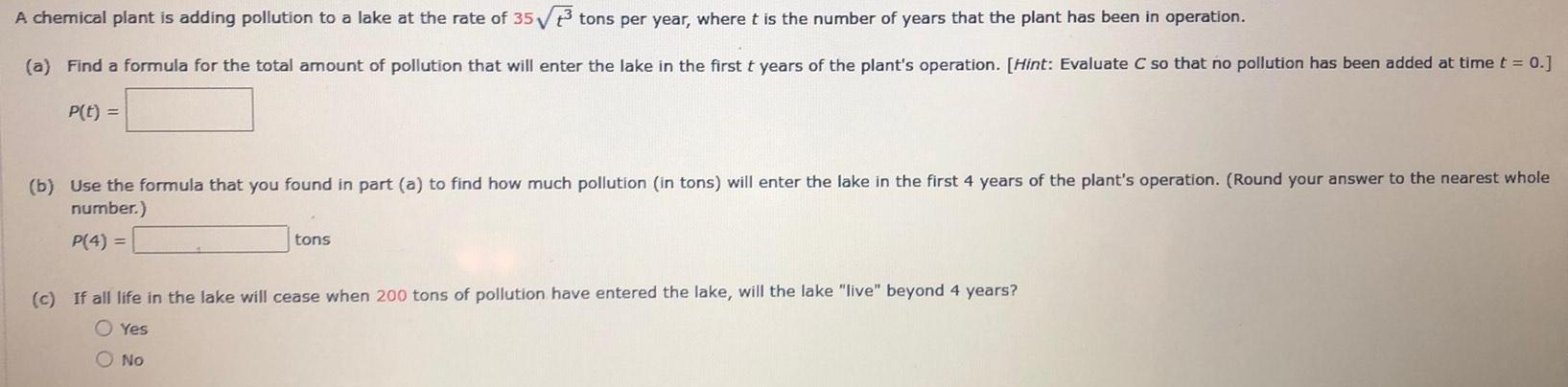Math
Differential equations
A chemical plant is adding pollution to a lake at the rate of 35√3 tons per year, where t is the number of years that the plant has been in operation. (a) Find a formula for the total amount of pollution that will enter the lake in the first t years of the plant's operation. [Hint: Evaluate C so that no pollution has been added at time t = 0.] P(t) = (b) Use the formula that you found in part (a) to find how much pollution (in tons) will enter the lake in the first 4 years of the plant's operation. (Round your answer to the nearest whole number.) P(4) = tons (c) If all life in the lake will cease when 200 tons of pollution have entered the lake, will the lake "live" beyond 4 years?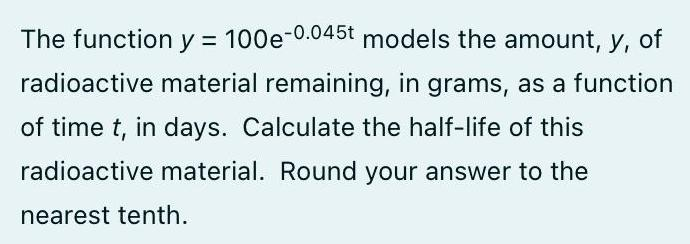Math
Differential equations
The function y = 100e^(-0.045t) models the amount, y, of radioactive material remaining, in grams, as a function of time t, in days. Calculate the half-life of this radioactive material. Round your answer to the nearest tenth.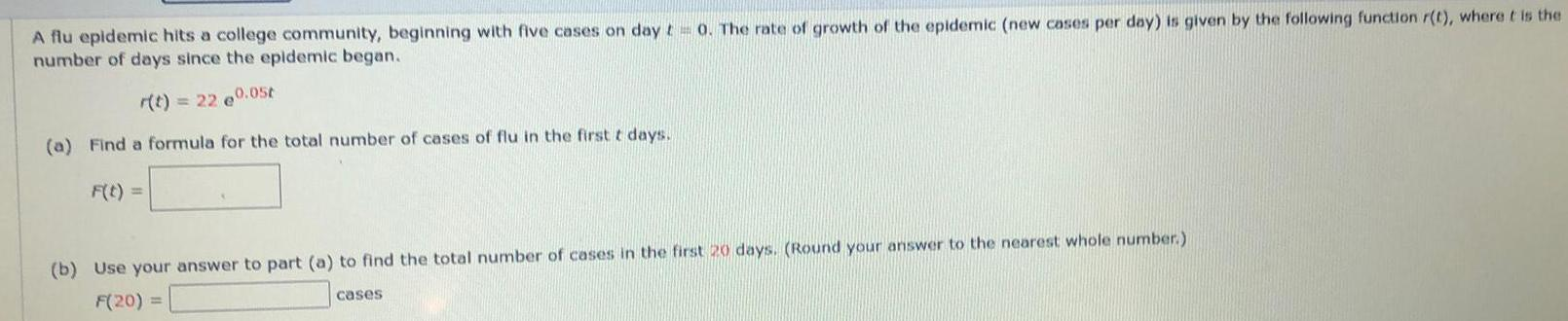Math
Differential equations
A flu epidemic hits a college community, beginning with five cases on day t = 0. The rate of growth of the epidemic (new cases per day) is given by the following function r(t), where it is the number of days since the epidemic began. r(t) = 22 e0.05t (a) Find a formula for the total number of cases of flu in the first t days. F(t) = (b) Use your answer to part (a) to find the total number of cases in the first 20 days. (Round your answer to the nearest whole number.) F(20) = cases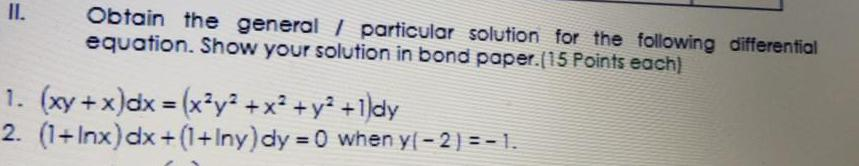Math
Differential equations
II. Obtain the general / particular solution for the following differential equation. Show your solution in bond paper.(15 Points each) 1. (xy + x) dx = (x³y² + x² + y² +1)dy 2. (1+Inx) dx+(1+Iny) dy =0 when y(-2) = -1.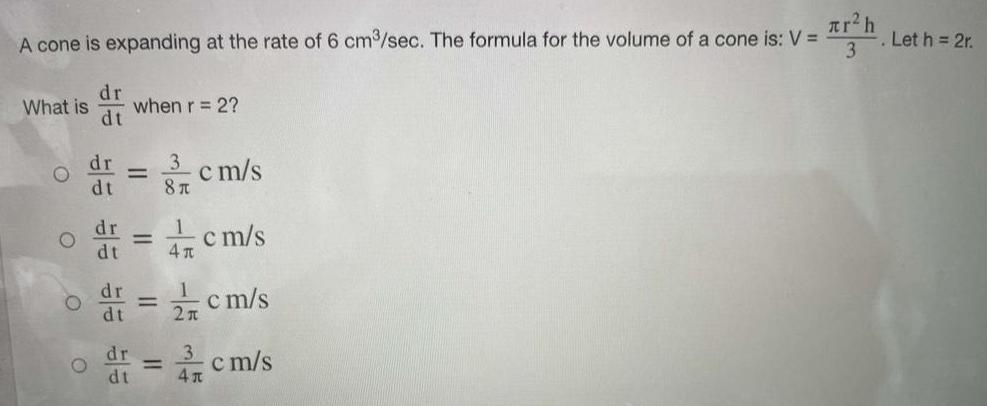Math
Differential equations
A cone is expanding at the rate of 6 cm³/sec. The formula for the volume of a cone is: What is when r = 2?